Univerza na Primorskem Fakulteta za matematiko, naravoslovje in informacijske tehnologije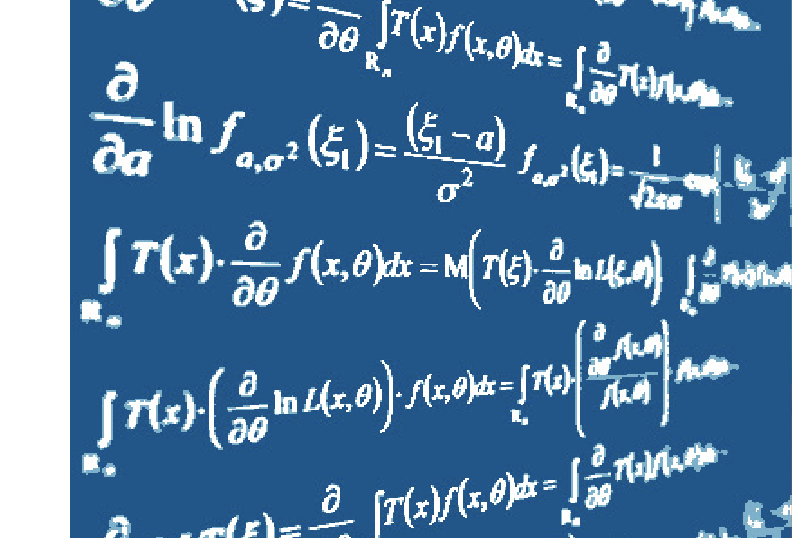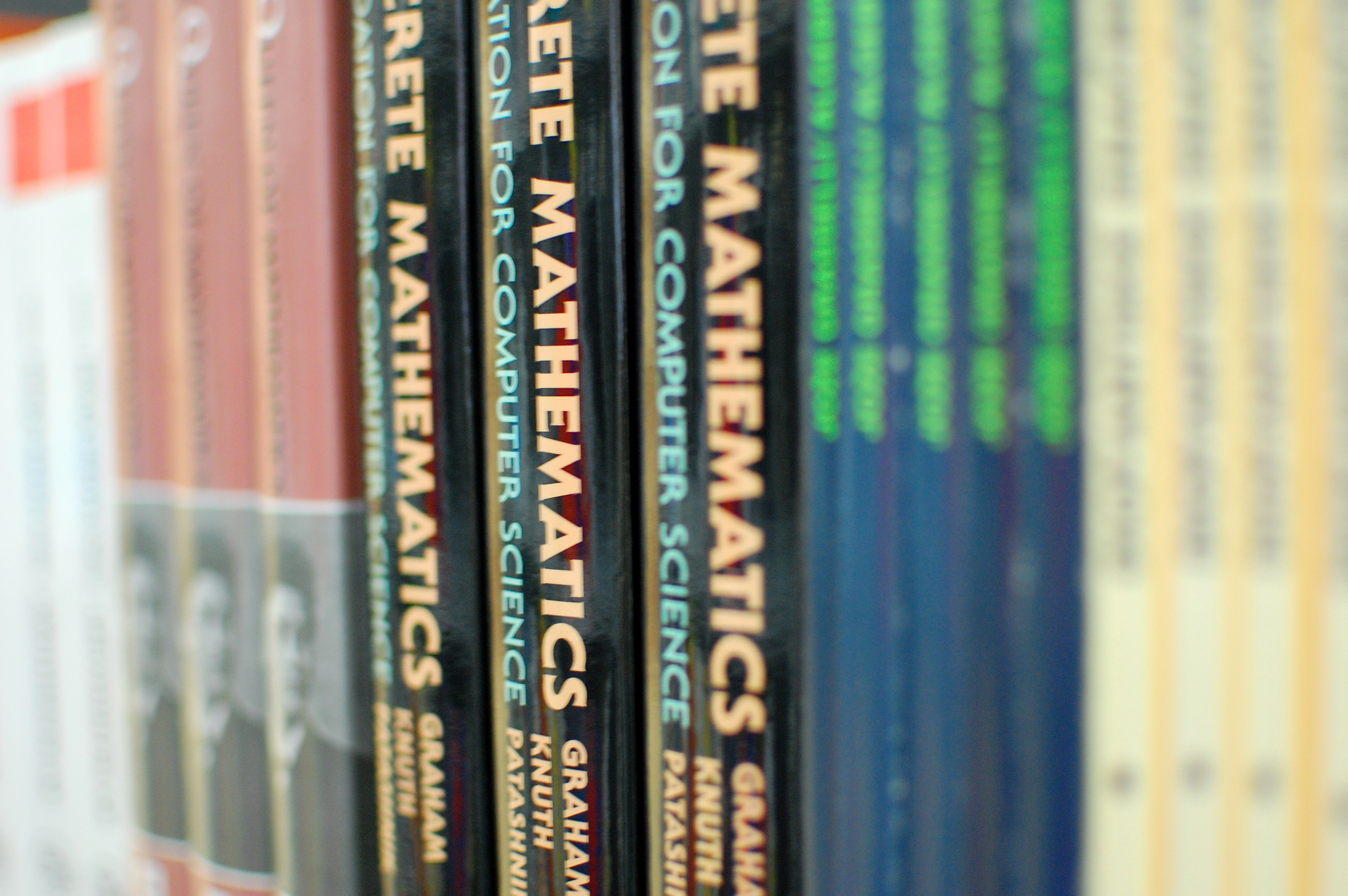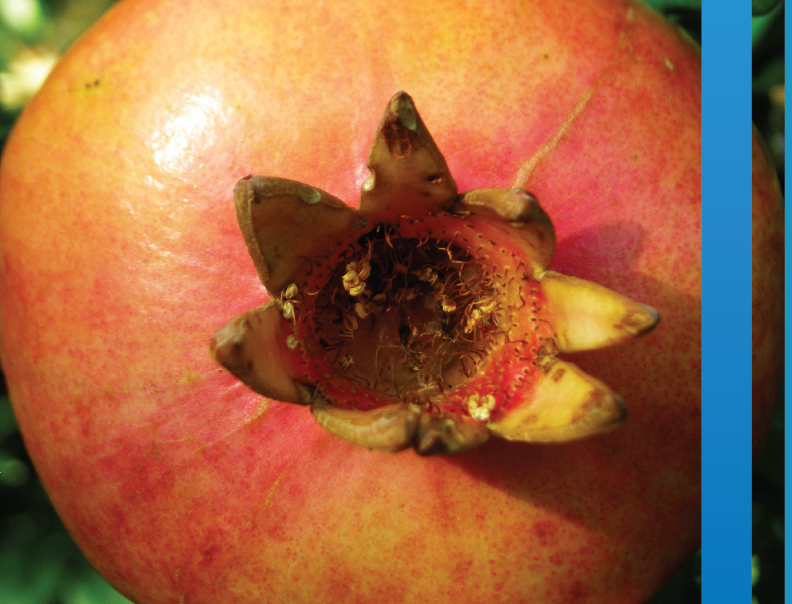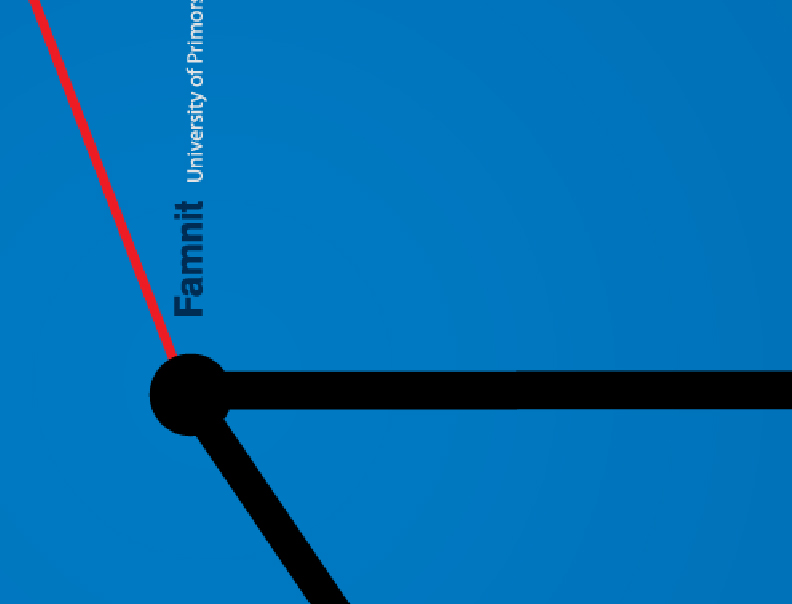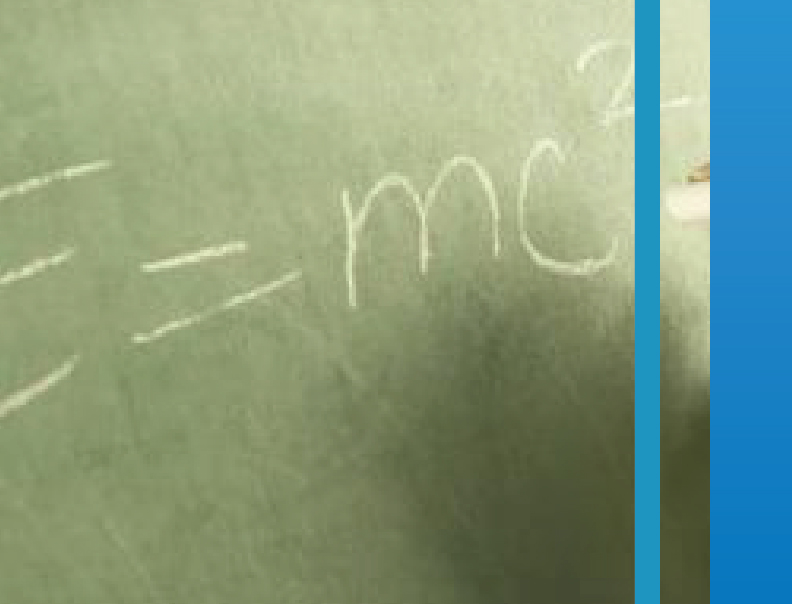SI | EN# Raziskovalni matematični seminar - Arhiv

 2020 2019 2018 2017 2016 2015 2014 2013 2012 2011 2010
 1 2 3 4 5 6 7 8 9 10 11 12

31.01.2011. ob 10:00 Seminarska soba v Galebu

Predavatelj: dr. Jiangtao Shi (School of Mathematical Sciences, Peking University, China)

Title: On an inverse problem to Frobenius's theorem

Abstract: Let G be a finite group and e a positive integer dividing |G|, the order of G.

Denoting Le(G) = {x \in G| xe = 1}, Frobenius proved that |Le(G)| = ke for a
positive integer k ≥ 1. In this talk, we give a complete classification of finite groups
G with |Le(G)| ≤ 2e for every e dividing |G|.

SLIDES FROM THE TALK ARE AVAILABLE HERE

10.01.2011. ob 10:00 Seminarska soba v Galebu
Predavatelj: dr. Bojan Kuzma

Naslov: On conversion of permanent into determinant.

Povzetek: Though definition of permanent looks deceptively similar to determinant, the former is much harder to calculate than the later. A possible remedy is to convert a matrix such that the permanent of original matrix equals determinant of converted matrix. This conversion is given by a map on matrices. We will show that for matrices over finite fields no such bijective conversion is possible.